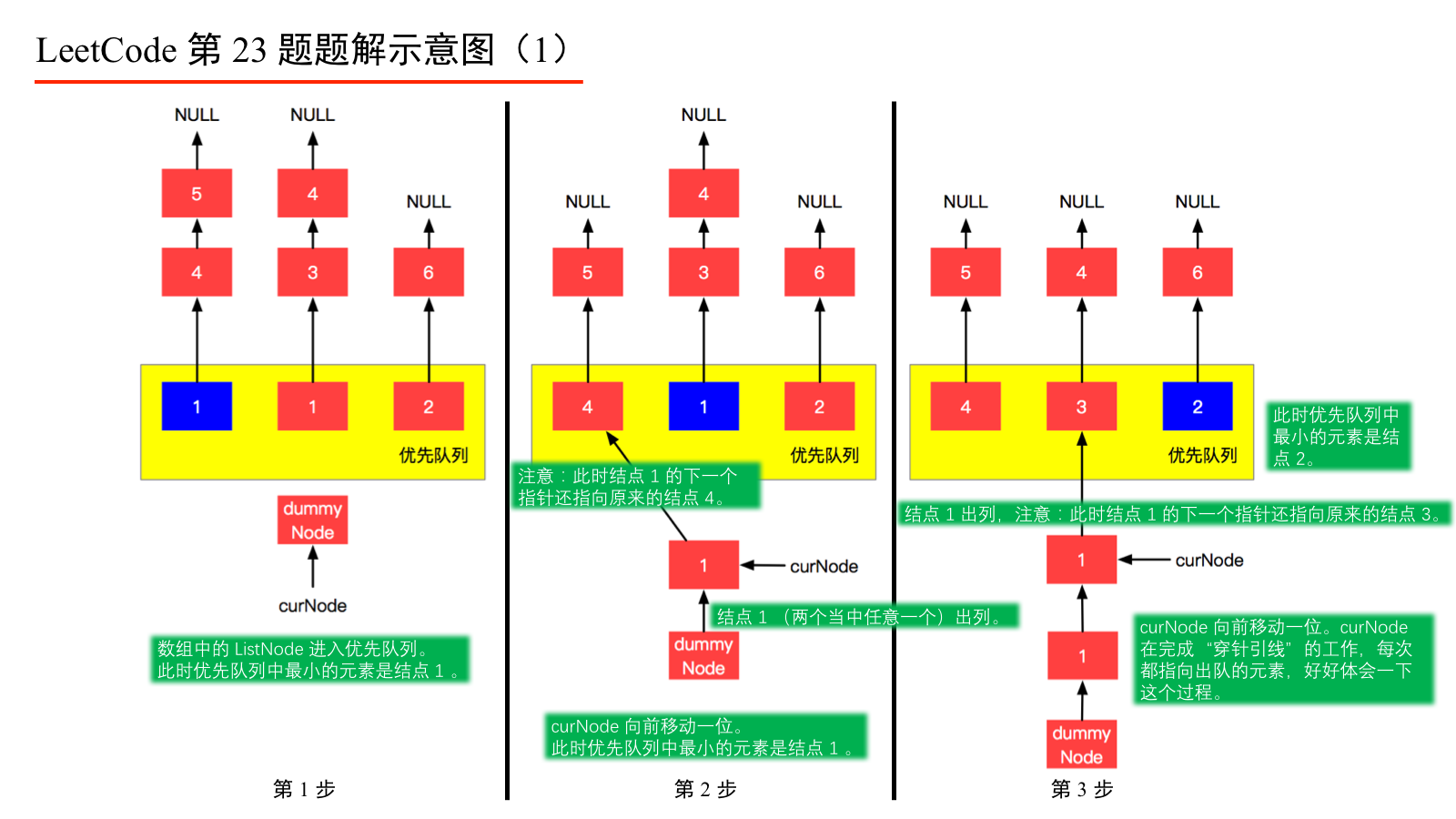# 23. Merge k Sorted Lists

• 题目描述：

```输入:
[
1->4->5,
1->3->4,
2->6
]

## 思路分析

（1）让三个班的学生按列站在你的面前，这时你能看到站在队首的学生的全身，其余同学只能看到比前面同学脑袋高出的那部分；

（2）每一次队首的 3 名同学，请出最矮的同学出列到“队伍4”（即我们最终认为排好序的队列），出列的这一列的后面的所有同学都向前走一步（其实走不走都行，只要你能比较出站在你面前的 3 位在队首的同学同学的高矮即可）；

（3）重复第（2）步，直到 3 个班的同学全部出列完毕。## 参考解答

### 参考解答1

``````import java.util.Comparator;
import java.util.PriorityQueue;

class ListNode {
int val;
ListNode next;

ListNode(int x) {
val = x;
}

ListNode(Integer[] nums) {
ListNode currNode = this;
currNode.val = nums;
for (int i = 1; i < nums.length; i++) {
currNode.next = new ListNode(nums[i]);
currNode = currNode.next;
}
}

@Override
public String toString() {
ListNode currNode = this;
StringBuilder s = new StringBuilder();
while (currNode != null) {
s.append(currNode.val);
s.append(" -> ");
currNode = currNode.next;
}
// 最后添加一个 NULL 标志表示添加到末尾了
s.append("NULL");
return s.toString();
}
}

public class Solution {

public ListNode mergeKLists(ListNode[] lists) {
int len = lists.length;
if (len == 0) {
return null;
}
PriorityQueue<ListNode> priorityQueue = new PriorityQueue<>(len, Comparator.comparingInt(a -> a.val));
ListNode dummyNode = new ListNode(-1);
ListNode curNode = dummyNode;
for (ListNode list : lists) {
if (list != null) {
// 这一步很关键，不能也没有必要将空对象添加到优先队列中
}
}
while (!priorityQueue.isEmpty()) {
// 优先队列非空才能出队
ListNode node = priorityQueue.poll();
// 当前节点的 next 指针指向出队元素
curNode.next = node;
// 当前指针向前移动一个元素，指向了刚刚出队的那个元素
curNode = curNode.next;
if (curNode.next != null) {
// 只有非空节点才能加入到优先队列中
}
}
return dummyNode.next;
}

public static void main(String[] args) {
Integer[] nums1 = {1, 4, 5};
Integer[] nums2 = {1, 3, 4};
Integer[] nums3 = {2, 6};
ListNode[] lists = new ListNode;
Solution solution = new Solution();
ListNode mergeKLists = solution.mergeKLists(lists);
System.out.println(mergeKLists);
}
}
``````

``````Comparator<ListNode> comparator = (a, b) -> a.val - b.val;
``````

``````Comparator<ListNode> comparator = Comparator.comparingInt(a -> a.val);
``````

### 参考解答2

``````class Solution2 {
public ListNode mergeKLists(ListNode[] lists) {
int len = lists.length;
if (len == 0) {
return null;
}
return mergeKLists(lists, 0, len - 1);
}

private ListNode mergeKLists(ListNode[] lists, int l, int r) {
// 思考这里为什么取等于？这是因为根据下文对 sort 的递归调用情况，区间最窄的时候，只可能是左右端点重合
if (l == r) {
return lists[l];
}
int mid = l + (r - l) / 2;
ListNode listNode1 = mergeKLists(lists, l, mid);
ListNode listNode2 = mergeKLists(lists, mid + 1, r);
// 于是问题转化成合并两个有序链表的问题了，我们可以穿针引线，也可以继续递归解决这个子问题，请见 LeetCode 第 21 题，
// 这里我们使用继续递归解决，
// 因为使用穿针引线，每一次调用这个方法的时候，都需要创建一个虚拟的头结点，归并次数有些多的时候，是不划算的
return mergeOfTwoListNode(listNode1, listNode2);
}

private ListNode mergeOfTwoListNode(ListNode listNode1, ListNode listNode2) {
// 先处理递归到底的情况
if (listNode1 == null) {
return listNode2;
}
if (listNode2 == null) {
return listNode1;
}
if (listNode1.val < listNode2.val) {
// 把问题转化为一个更小的问题
listNode1.next = mergeOfTwoListNode(listNode1.next, listNode2);
return listNode1;
} else {
// 把问题转化为一个更小的问题
listNode2.next = mergeOfTwoListNode(listNode1, listNode2.next);
return listNode2;
}
}
}
``````